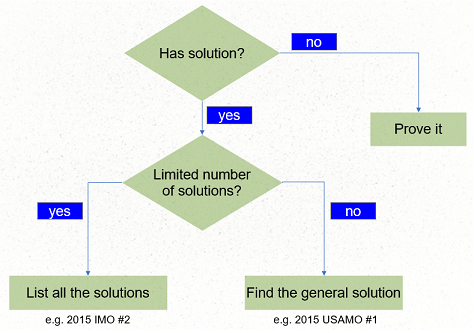Introduction Default

Video tutorial

Lecture Notes

As its name suggests, an indeterminate equation usually has an indetermined number of, i.e. infinitely many, solutions. But usually we are only interested in its integer solutions. Therefore, solving an indeterminate equation means to find its (positive? non-negative? any) integer solutions.While some indeterminate equations can be very challenging to solve (this is why such problems appear in IMO/AMO levels), most indeterminate equations in entry to intermediate level contests are reasonably easy if you can recognize their pattern. Hence, the focus of learning indeterminate equation is on learning various well-known patterns.